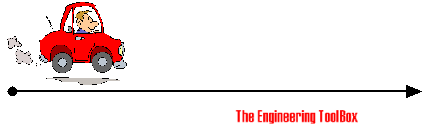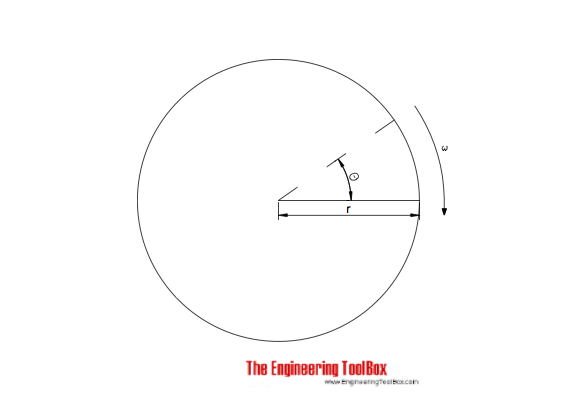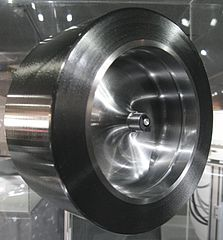Engineering ToolBox - Resources, Tools and Basic Information for Engineering and Design of Technical Applications!

# Formulas of Motion - Linear and Circular

## Linear and angular (rotation) acceleration, velocity, speed and distance.

### Linear Motion FormulasAverage velocity/speed of a moving object can be calculated as

v = s / t                            (1a)

where

v = velocity or speed (m/s, ft/s)

s = linear distance traveled (m, ft)

t = time (s)

• distance is the length of the path a body follows in moving from one point to another - displacement is the straight line distance between the initial and final positions of the body
• we use velocity and speed interchangeable - but be aware that speed is a measure of how fast or slow a distance is covered, the rate at which distance is covered - velocity is a vector, specifying how fast or slow a distance is covered and the direction

If acceleration is constant then velocity can be expressed as:

v = v0 + a t                            (1b)

where

v0 = initial linear velocity (m/s, ft/s)

a = acceleration (m/s2, ft/s2)

Linear distance can be expressed as (if acceleration is constant):

s = v0 t + 1/2 a t2                                       (1c)

Combining 1b and 1c to express the final velocity

v = (v02 + 2 a s)1/2                                    (1d)

Velocity can be expressed as (velocity is variable)

v = ds / dt (1f)

where

ds = change in distance (m, ft)

dt = change in time (s)

Acceleration can be expressed as

a = dv / dt                                   (1g)

where

dv = change in velocity (m/s, ft/s)

#### Example - a Marathon Run

If a marathon - 42195 m - is run in amazing 2:03:23 (7403 seconds) (Wilson Kipsang, Kenya - September 29, 2013 Berlin Marathon) - the average speed can be calculated

v = (42195 m) / (7403 s)

= 5.7 m/s

= 20.5 km/h

#### Example - Acceleration of a Car

A car accelerates from 0 km/h to 100 km/h in 10 seconds. The acceleration can be calculated by transforming (1b) to

a = (v - v0) / t

= ( (100 km/h) (1000 m/km) / (3600 s/h) - (0 km/h) (1000 m/km) / (3600 s/h) ) / (10 s)

= 2.78 (m/s2)

### Linear Motion Calculators

#### Average velocity

s - distance (m, km, ft, miles)

t - time used (s, h)

#### Distance

v0 - initial velocity (m/s, ft/s)

a - acceleration (m/s2, ft/s2)

t - time used (s, h)

#### Final Velocity

v0 - initial velocity (m/s, ft/s)

a - acceleration (m/s2, ft/s2)

s - distance (m, ft)

#### Acceleration

v - final velocity (m/s, ft/s)

v0 - initial velocity (m/s, ft/s)

t - time used (s)

### Circular Motion - Rotation#### Angular Velocity

Angular velocity can be expressed as (angular velocity = constant):

ω = θ / t                           (2)

where

t = time (s)

Angular velocity and rpm:

ω = 2 π n / 60                        (2a)

where

n = revolutions per minute (rpm)

π = 3.14...

The tangential velocity of a point in angular velocity - in metric or imperial units like m/s or ft/s - can be calculated as

v = ω r                              (2b)

where

v = tangential velocity (m/s, ft/s, in/s)

r = distance from center to the point (m, ft, in)

#### Example - Tangential Velocity of a Bicycle Tire

A 26 inches bicycle wheel rotates with an angular velocity of π radians/s (0.5 turn per second). The tangential velocity of the tire can be calculated as

v = (π radians/s) ((26 inches) / 2)

= 40.8 inches/s

#### Angular Velocity and Acceleration

Angular velocity can also be expressed as (angular acceleration = constant):

ω = ωo + α t                               (2c)

where

ωo = angular velocity at time zero (rad/s)

α = angular acceleration or deceleration (rad/s2)

#### Angular Displacement

Angular distance can be expressed as (angular acceleration is constant):

θ = ωo t + 1/2 α t2                                     (2d)

Combining 2a and 2c:

ω = (ωo2 + 2 α θ)1/2

#### Angular Acceleration

Angular acceleration can be expressed as:

α = dω / dt = d2θ / dt2                                    (2e)

where

dθ = change of angular distance (rad)

dt = change in time (s)

##### Example - Flywheel DecelerationBy Geni (Photo by User:geni) [GFDL or CC-BY-SA-3.0-2.5-2.0-1.0], via Wikimedia Commons

A flywheel is slowed down from 2000 rpm (revolutions/min) to 1800 rpm in 10 s. The deceleration of the flywheel can be calculated as

α = ((2000 rev/min)  - (1800 rev/min)) (0.01667 min/s) (2 π rad/rev) / (10 s)

= 120 degrees/s2

#### Angular Moment - or Torque

Angular moment or torque can be expressed as:

T = α I                                 (2f)

where

T = angular moment or torque (N m)

I = Moment of inertia (lbm ft2, kg m2)

## Related Topics

• ### Dynamics

Motion - velocity and acceleration, forces and torque.
• ### Mechanics

Forces, acceleration, displacement, vectors, motion, momentum, energy of objects and more.

## Related Documents

• ### Acceleration

Change in velocity vs. time used.
• ### Acceleration Units Converter

Converting between units of acceleration.
• ### Angular Motion - Power and Torque

Angular velocity and acceleration vs. power and torque.
• ### Banked Turn

A turn or change of direction in which the vehicle banks or inclines, usually towards the inside of the turn.
• ### Car - Required Power and Torque

Power, torque, efficiency and wheel force acting on a car.
• ### Car Acceleration

Car acceleration calculator.
• ### Centripetal and Centrifugal Acceleration Force

Forces due to circular motion and centripetal / centrifugal acceleration.
• ### Conn-Rod Mechanism

The connecting rod mechanism.
• ### Conservation of Momentum

The momentum of a body is the product of its mass and velocity - recoil calculator.
• ### Disk Brakes - Torque and Force

Forces and torque activated with disk brakes.
• ### Distance Traveled vs. Speed and Time - Calculator and Chart

Velocity plotted in time used diagram.
• ### Elevators - Force and Power

Required force and power to lift an elevator.
• ### Flywheels - Kinetic Energy

The kinetic energy stored in flywheels - the moment of inertia.
• ### Impulse and Impulse Force

Forces acting a very short time are called impulse forces.
• ### Kinetic Energy

Energy possessed by an object's motion is kinetic energy.
• ### Piston Engines - Displacement

Calculate piston engine displacement.
• ### Projectile Range

Calculate the range of a projectile - a motion in two dimensions.

Radian is the SI unit of angle. Convert between degrees and radians. Calculate angular velocity.
• ### Rolling Resistance

Rolling friction and rolling resistance.
• ### Vehicle - Distance Traveled vs. Velcocity and Time Used (mph)

Speed (mph) and time (hours) and distance traveled (miles) chart.
• ### Vehicle - Distance Traveled vs. Velocity and Time (km/h)

Speed (km/h) vs. time (hours) and distance traveled (km).
• ### Velocity Units Converter

Convert between speed and velocity units like m/s, km/h, knots, mph and ft/s.

## Engineering ToolBox - SketchUp Extension - Online 3D modeling!

Add standard and customized parametric components - like flange beams, lumbers, piping, stairs and more - to your Sketchup model with the Engineering ToolBox - SketchUp Extension - enabled for use with older versions of the amazing SketchUp Make and the newer "up to date" SketchUp Pro . Add the Engineering ToolBox extension to your SketchUp Make/Pro from the Extension Warehouse !

We don't collect information from our users. More about

## Citation

• The Engineering ToolBox (2005). Formulas of Motion - Linear and Circular. [online] Available at: https://www.engineeringtoolbox.com/motion-formulas-d_941.html [Accessed Day Month Year].

Modify the access date according your visit.

9.19.12